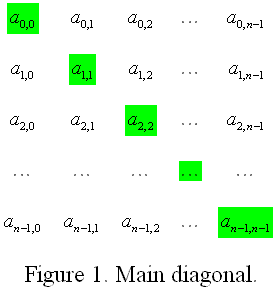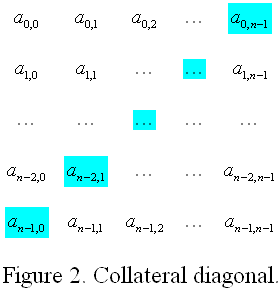### Problem Statement

Note: this problem statement contains images that may not display properly if viewed outside the applet.

You are to calculate the diagonal disproportion of a square matrix. The diagonal disproportion of a square matrix is the sum of the elements of its main diagonal minus the sum of the elements of its collateral diagonal. The main and collateral diagonals of a square matrix are shown in figures 1 and 2 respectively.The elements of the main diagonal are shown in green in figure 1, and the elements of the collateral diagonal are shown in cyan in figure 2.

Given a String[] matrix, return its diagonal disproportion. The j'th character of the i'th element of matrix should be treated as the element in the i'th row and j'th column of the matrix.

### Definition

 Class: DiagonalDisproportion Method: getDisproportion Parameters: String[] Returns: int Method signature: int getDisproportion(String[] matrix) (be sure your method is public)

### Constraints

-matrix will contain between 1 and 50 elements, inclusive.
-Each element of matrix will contain only digits ('0'-'9').
-The number of characters in each element of matrix will be equal to the number of elements in matrix.

### Examples

0)

 `{"190","828","373"}`
`Returns: 1`
 The sum of the elements of the main diagonal is 1+2+3 = 6. The sum of the elements of the collateral diagonal is 0+2+3 = 5. So, the answer is 6-5 = 1.
1)

 `{"9000","0120","0000","9000"}`
`Returns: -1`
2)

 `{"6"}`
`Returns: 0`
 The matrix has only one element, and this element lies on both the main and collateral diagonals.
3)

 ```{"7748297018","8395414567","7006199788","5446757413","2972498628", "0508396790","9986085827","2386063041","5687189519","7729785238"}```
`Returns: -24`

#### Problem url:

http://www.topcoder.com/stat?c=problem_statement&pm=6003

#### Problem stats url:

http://www.topcoder.com/tc?module=ProblemDetail&rd=8080&pm=6003

gevak

#### Testers:

PabloGilberto , brett1479 , Olexiy

#### Problem categories:

Simple Search, Iteration